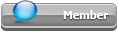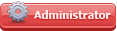## Featured Articles

Check out the latest featured articles.## File Library

Check out the latest downloads available in the File Library.0# Optimization Problem, Minimize Heat Exchanger Area

optimization matlab heat exchangers minimize minimum fmincon

2 replies to this topic
|

### #1newbieneedshelp

newbieneedshelp

Brand New Member

•• Members
•• 2 posts

Posted 21 February 2022 - 07:13 AM

I want to solve the following optimization problem from Optimization of Chemical processes:

Here is what I have done so far:

- Assume wcp is constant for all streams

- Contraints can be derived from the equations of heat: Q=wcp*dTcold=wcp*dThot=U*A*dTLM

Where dTcold & dThot are the change in temperature of each cold and hot stream in a heat exchanger. And dTLM=(dT1-dT2)/ln(dT1/dT2).

A clearer representation of this can be seen in the following image:

- With that the objective function is specified as: Atotal = A1+A2+A3

- Some linear equilibrium constraints can be made as follows:

From the heat equation dTcold=dThot, from that I got:

T1+T5=400

-T1+T2+T4=400

-T2+T3=100

-The inequality linear constraints can be set as:

T1<T2

T2<T3

T1<T4

-Lower and upper bounds for the variables are somewhat irrelevant, given the constraints, either way I specified them as:

0<A1,2,3<Inf

100<T1<300

100<T2<400

100<T3<600

100<T4<400

100<T5<300

-Lastly, some non-linear constraints were specified as:

U*A*dTLM-wcp*dTcold=0

Could have used dTcold or dThot, but should be the same if the other contraints hold.

Coded that into Matlab (and I have checked multiple times that everything is coded as described above), but I get very inconsistent results, a slight change in the initial guess of the estimated parameters leads to a different answer every time. Is there something wrong with my approach?

### #2breizh

breizh

Gold Member

••• 5,562 posts

Posted 21 February 2022 - 09:29 AM   Best Answer

Hi,

Did you try Excel and solver ?

Your set of equations seems correct .

Initialization step :T1,T2,T3,T4,T5

Calculation step :  Q1 ,Q2,Q3  then  A1 ,A2,A3

Optimization step  Min (A1+A2+A3) by changing T1,T2,T3,T4,T5 with associated constraints .

Good luck

Breizh

### #3Bobby Strain

Bobby Strain

Gold Member

•• Members
•• 3,272 posts

Posted 21 February 2022 - 12:03 PM

You have only two variables. So it's simple enough to use trial and error. Track overall area, then pick the smallest. Best if you use VBA. Better still, code it in VB.NET or C#.

Bobby